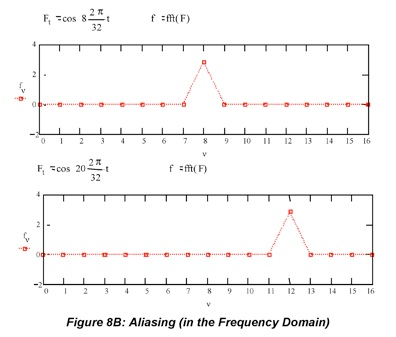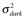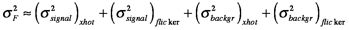8. Discrete FT & NoiseDiscrete Fourier transformation

Experimental data procesed by a computer is comprised of discrete points; therefore, the Fourier transforms used on experimental data are always discrete Fourier transforms.  Before we look at the expressions for the discrete transform it is important to see how digitization impacts experimental analog signals.  Figure 8A illustrates the digitization of a continuous, analog single frequency interferogram.  This figure shows that digitization multiplies the analog signal by a sequence of evenly spaced Dirac pulses (the sampling function).  The result is a sequence of N evenly space points that represent the analog signal: {f(t0), f(t1), … , f(tN-1)}. When a sequence is digitized, its Fourier transform is very similarto its continuous counterpart.  The principle difference is in the fact that the sequence and its transform are vectors and the indexing has important unique features. (There is a wide variety of commercial software for calculating the Fourier transform of a digitized waveform numerically. Many of these programs are based upon the Cooley-Tukey algorithm, which is an efficient, timesaving way of programming the Fourier transform, and is often called the "Fast Fourier transform algorithm". Using any personal computer, a Fast Fourier transform (FFT) on 1024 data points takes just a few seconds.) The traditional FFT requires that the waveform (digital sequence) have 2n data points, where n is any integer. Others do not, but in either case the calculated spectrum has only half the number of unique data points as the time-domain function.  This arises from the fact that simple transformation does not distinguish between positive and negative frequencies. Remember that cos(ω0) and cos(-ω0) are identical so the positive and negative cosine (real) Fourier coefficients have the same amplitudes. In the case of the sines, the signs are different. Formally this is reflected in the Nyquist criterion, which states that a waveform of frequency, ω, can only be characterized by sampling the waveform at a rate of at least 2ω.  Hence the number of unique frequencies in the spectrum is a factor of 2 smaller than the number of points in the time domain sequence. The discrete FT of a sequence f(tn) iswhere the frequency index m steps from –N/2 to N/2 to reflect the reduction of the number of unique points in the transform.

The Nyquist criterion has another important consequence in computing transforms of discrete sequences: whenthe sampling rate is too low, signal components that have frequencies above the Nyquist frequency are “aliased” (folded) into lower frequencies. The example in Figure 8B shows what happens when the frequency of a single frequency interferogram exceeds 1/(2Δt), where 1/(2Δt) is the highest frequency the Fourier transform can compute and Δt is the time elapsed between sampled points. This re-states the Nyquist criterion: if 1/Δt is the sampling frequency, then 1/2Δt is the maximum frequency observed without aliasing.   The highest frequency in the example transform is 2π(16/32) so the spectrum at ω=2π(8/32) is represented correctly, but a spectrum at ω=2π(20/32), which should be outside the frame, is misrepresented at ωapparentsamplingtrue=2π(12/32).  When the frequency content of a waveform is high, the time axis has to have points sufficiently close together to capture the high frequency features. Otherwise each high frequency component appears as if it has a lower frequency in the transform. This isillustrated in Figure 8C, where the same cosine wave is plotted with a time axis that has points close together (red) vs. far apart (blue). Sampling the high frequency wave with too few points makes it appear to be a lower frequency wave.   Here the “free spectral range’ (FSR) describes the range of frequencies that can be sampled without aliasing: FSR=1/2Δt.

Now that the signals have been digitized, we can discuss transformation and convolution as a series of sums rather than integrals. In practice most convolutions are carried out by computing the Fourier transforms, multiplying them, then computing the inverse transform of the product, but it is easiest to understand what convolution does in the time domain.  Convolution is a type of vector multiplication that blends the features of the two sequences.  This operation occurs often in nature:  when a simple camera captures an image of a large object (see http://www.vias.org/tmdatanaleng/… cc_convolution.html for a humorous, but effective cartoon), a telescope captures images of the sky, an AFM (atomic force microscopy) tip images a surface and when a source is dispersed into a spectrum by a monochromator equipped with entrance and exit slits.  We will consider a simplified version of this last example.  Consider the sequence f=[0 1 2 1 0] to represent a spectrum and the sequence h=[0 1 1 1 0] to represent the exit slit of a monochromator. The spectrum is recorded at the detector as the grating is turned and the wavelengths that pass through the slit change. The discrete convolution is given by the expressionThe total number of points in a discrete convolution is one more than the combined number of points in the two sequences.  The convolution of the two sequences is g=[ 0 0 1 3 4 3 1 0 0 ]. Technically, each element of the convolution is a sum of nine terms. (See a graphical representation of seven of them at this link.) Since most of them are zero, it is useful to only consider the indices that correspond to the spectrum points that are in front of the slit.  We do this by changing the summation to cover the relative units around the center of the spectrum k-i.  In this example k-i steps from -2 to 2.  So the first non-zero element of the convolution occurs when i=3.  At this grating setting, the last non-zero element of the “spectrum” overlaps the first non-zero element of the “slit”, so the intensity recorded at the detector, g(i), is 1. The spectrum was broadened by passing through the slit because the slit is not infinitely narrow (a Dirac) as it would need to be to form a perfect 1:1 image.

In discrete processing, the truncation of signals by finite acquisition times is an important application of convolution, too. Again we treat the data set as though it were an infinite function multiplied by a window (rectangle) function.  (Remember the window function is defined as unity at the time points when the data set is measured and zero outside of the data set).  As previously discussed, the Fourier transform of a rectangle is a sinc function.  So when a 1024 point cosine wave is truncated to 300 data points, the transform is the convolution of the transform of the waveform (Dirac function) and the transform of the window function (sinc function). This is illustrated below in Figure 8D.Now we see why even very narrow spectral bands will appear as sinc functions that have widths inversely proportional to the mirror travel (the width of the window) in the FTIR spectrum.  We also see why the spectral resolution of the FTIR instrument is controlled by the mirror travel, Δz.  The exact relation between the conjugate variables mirror travel, Δz, and spectral bandwidth (think FWHM), Δk, is 2πΔk=1.2/Δz.  Most textbooks, including I&C, approximate 1.2 as 1 to simplify the calculation.
The oscillations in the baseline of the sinc function are undesirable in spectroscopy because they can distort neighboring resonances.  The oscillation can be suppressed by “apodization”, in which the interferogram is multiplied by amonotonically decaying function such as a Gaussian or an exponential in order to attenuate the oscillation in the baseline.  The example in Figure 8E shows that multiplication of the truncated cosine wave by an exponential gives a Lorentzian profile.  Apodization always lowers the resolution, but its benefits often outweigh its disadvantages.This trade-off between resolution and baseline stability has another important consequence when the FT is used to compute the spectrum from a time domain waveform because the number of waveform points determines the number of frequencies that can be represented in the spectrum.  Since transformation cuts the number of points used to represent the spectrum, computing the transform of a waveform to which N zeros have been appended increases the number of unique frequencies to equal the number of waveform points.  This does not increase the frequency content of the spectrum, remember this is determined by the Nyquist frequency (1/2Δt), but it increases the number of points used to represent the frequency axis.  The addition of N points to the waveform can improve the resolution of a spectrum, as shown in Figure 8F.  Adding more than N zeros doesn’t add any additional information to the spectrum, but it can make small shoulders more visible.  However, this comes at the cost of decreased baseline stability.  This trade-off is clearer in the original figure, which you can find in Figure 3.7 of Marshall & Verdun on page 81.

Noise

In experimentation, there is always noise. Noise consists of random fluctuations superimposed on the analytical signal. Noise is characterized by its magnitude, its frequency spectrum and/or its source.  The magnitude of noise can be measured in terms of signal variance, σ2, when measurement errors are normally distributed or in terms of counts, n, in spectroscopic measurements described by counting (Poisson) statistics.  In both cases, the signal to noise ratio is a convenient measure.  Expressions for some of these parameters arewhere Φi represents the radiant power measured at each point in the measurement, Ni represents the number of photons counted at each point in the measurement and n represents the total number of data points collected in the measurement.

How much noise is superimposed on the signal depends on several factors especially the frequency at which the measurements are made.  Figure 8G depicts the relative amount of noise observed from various sources in a university laboratory.  Notice in particular how noisy the low frequency range, i.e., where steady state measurements are made, is.  Herein lies the value of modulation based strategies, such as lock-in amplification, which move the measurement to a quiet frequency region where environmental noise is lower.  Various instruments have similar noise spectra revealing thenature of the noise impacting the measurement process.  For example the noise power spectrum of a fluorescence spectrometer, would look similar to Figure 8G.  The low frequency noise of such instruments is large because frequency dependent 1/f or flicker noise is a major noise source in fluorescence measurements.  Of course, there should not be such large interference bands since the instrument components should be insulated to minimize pick-up of environmental noise. However, there can be exceptions, for example large NMR spectrometers nearby (e.g., next door) can produce significant interference noise in the RF frequency range.  The noise power spectrum of detector noise limited instruments, such as IR spectrometers, is essentially flat because it is dominated by frequency independent white noise such as shot and thermal (Johnson) noise.
Variances are additive so they can be partitioned according to their source.  For example, most spectroscopic signals are degraded by noise in the analytical signal, background signal and detector (dark) signal.  Each of these variances can be partitioned further to reflect the circumstances of the measurement.  For example, the noise in IR absorption measurements is dominated by fundamental and thermal noise from the detector because IR sources are typically weak sowhereis the detector dark noise and(ar stands for amplifier read-out) is the thermal noise impacting the detection electronics.  On the other hand, fluorescence measurements are distorted by flicker (1/f) noise from the source in the signal and the background as well as fundamental (signal shot and quantum) noise in both these components, so the variance isIn fact, the background can be so large in these measurements that it is more useful to measure and compare S/B rather than S/N.  Ingle & Crouch give a very thorough treatment of this topic that can be difficult to read in some places.  Read that material with a view to extract the definitions and descriptions of how noise is generated in instrument components.

In some cases it is enough to measure the noise and report its magnitude with the results, but more often we analyze the noise because we are looking for ways to reduce it.  There are essentially two ways to do this.  In the first, we use hardware or electronic components such as electronic (LRC) filters, photon counting electronics or lock-in amplifiers to suppress the high-frequency noise in the measured signal.  In the second, we use digital filters (computer algorithms) to reduce the noise in the signal after it has been collected.  Fourier mathematics play a role in this second strategy because signals and noise are frequently characterized by their frequency content.  In most measurements, spectral information primarily consists of low frequency components.  If the noise is white noise, as is the case in detector noise limited measurements, it consists of (roughly) equal amounts of noise at all frequencies.  In this case, filtering the higher frequency components of the signal improves the signal-to-noise ratio of the data because the signal is mostly unaffected. Fourier transforms can facilitate this process, but the same concerns that guide choices in sampling and apodization apply here. The high frequency components of the signal are removed by multiplying the transform of the data (not the inverse transform, even if the data is a spectrum) by a smoothing function that attenuates the high frequency components.  The simplest smoothing function is the rectangle or window function.  All the frequencies above some user selected cut off frequency are set to zero.  The problem with this is that a sinc function can introduce ripples to the baseline of the spectrum as the last panel of Figure 8H illustrates.  More often filters employ Gaussian or other smoothly decaying functions, which eliminate the high frequency components without introducing the unstable baseline.Filters do not always improve the quality of spectral data.  Measurements that are dominated by noise from the source, e.g., fluorescence, are often distorted by flicker noise.  Whereas white noise is frequency independent, flicker noise is larger at low frequencies.  In fact, it is sometimes called pink noise (since low frequencies are associated with red rather than blue light).  Filtering techniques are based on the idea that the noise does not, in general, consist of the same frequency components as the signal.  In the case of flicker noise, this condition is not met.  This is one reason that FT-UV-VIS spectrometers are not widely used.  The improvement in signal to noise that comes from measuring the entire spectrum many times using an interferometer during the time a single spectrum can be measured on a dispersive (monochromator based) spectrometer is the square root of the number of times the spectrum was measured for shot noise limited (FT-IR) measurements.  In other words, a ten point spectrum measured by the interferometer has a signal to noise ratio that is about three times () larger than the same spectrum measured using a dispersive device because signal averaging reduces random noise by the square root of the number of acquisitions. (Remember this is called the multiplex or Fellgett advantage.)  This improvement is always smaller in the presence of flicker noise, and in very crowded spectra, a multiplex disadvantage in which the signal-to-noise ratio in the data acquired by the interferometer is lower than in the dispersive instrument can be observed.

(8.1)

(8.2)

(8.3a)

(8.3b)

(8.3c)

(8.4)

Last Updated: 4/07/10